## Chapter 1General results from representation theory

### 1.1. Basic definitions

Let G be a finite group. A representation of G on a vector space V is a pair (π,V ) where V is a complex vector space and π is a homomorphism G GL(V ). Often, we will denote (π,V ) simply by π, specially when the vector space V is specified implicitly. The dimension of V is called the degree of the representation (π,V ). In these notes all representations will be assumed to be of finite degree. If (π,V ) and (τ,U) are two representations of G, then a linear map φ : U V is called a homomorphism of G-modules, or an intertwiner if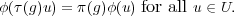The space of all homomorphisms (τ,U) (π,V ) will be denoted by HomG(τ,π). When φ is invertible, it is an isomorphism, and we say that τ is isomorphic to φ. The representations π and τ are said to be disjoint if HomG(τ,π) = 0.

### 1.2. The Pontryagin dual of a finite abelian group

Let G be an abelian group. The binary operation on the group will be written additively. A character of G is a homomorphism χ : G C*. In other words, χ(x + x) = χ(x)χ(x) for all x,x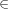G. A character χ is called unitary if χ(x)= 1 for all xG.

Exercise 1.1. Show that every character of a finite abelian group is unitary.

If G is a finite abelian group, its Pontryagin dual is the set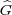of its characters. Under point-wise multiplication of characters,forms a group. Once again, the binary operation is written additively, so that given characters χ and χof G, (χ + χ)(x) = χ(x)χ(x) for all xG. This is a special case of a general construction for locally compact abelian groups.

Proposition 1.2. For any finite abelian group G, G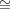.

Proof. The proof is a sequence of exercises:

Exercise 1.3. Show that the Proposition is true for a finite cyclic group Z∕nZ.

Exercise 1.4. If G1 and G2 are abelian groups, show that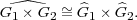Exercise 1.5. Show that every finite abelian group is isomorphic to a product of finite cyclic groups.

It follows from the above proposition that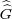G. However, in this case, there is a canonical isomorphism G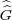given by gǧ where ǧ is defined by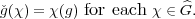### 1.3. Induced Representations

Let H be a subgroup of G. Given a representation (π,V ) of H, the representation of G induced from π is the representation (πG,V G) where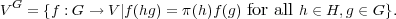The action of G on such functions is by right translation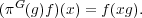Now suppose that (τ,U) is a representation of G and (π,V ) is a representation of H. Because H G, we can regard U as a representation of H by restricting the homomorphism G GL(U) to H. Denote this representation by τH. Given φHomG(τ,πG), define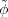: U V by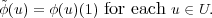Exercise 1.6. Show thatHomH(τH).

Theorem (Frobenius reciprocity). The map φinduces an isomorphism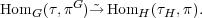Proof. For ψHomH(τH) define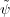: U V G by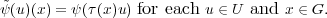Exercise 1.7. For all hH,(u)(hx) = π(h)(u)(x). Therefore,(u)V G.

Exercise 1.8. Show that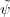HomG(τ,πG).

Exercise 1.9. For all φHomG(τ,πG),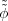= φ, and for all ψHomH(τH),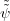= ψ.

Therefore the maps φand ψare mutual inverses.

### 1.4. Description of intertwiners

In this section we describe the homomorphisms between two induced representations. Let G be a finite group. Let H1 and H2 be subgroups. Let (π1,V 1) and (π2,V 2) be representations of H1 and H2 respectively. For f : G V 1, and Δ : G HomC(V 1,V 2), define a convolution Δ * f : G V 2 by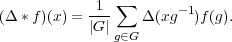Let D be the set of all functions Δ : G HomC(V 1,V 2) satisfying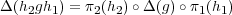for all h1H1, h2H2 and gG.

Exercise 1.10. Show that if ΔD and f1V 1G then Δ*f1V 2G.

Exercise 1.11. Show that the map LΔ : V 1G V 2G defined by f1Δ * f1 is a homomorphism of G-modules.

Theorem 1.12 (Mackey). The map ΔLΔ is an isomorphism from D HomG(V 1G,V 2G).

Proof. We construct an inverse mapping HomG(V 1G,V 2G) D. For this, let us define a collection fg,v of elements in V 1G indexed by gG and vV 1: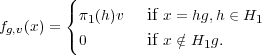Exercise 1.13. Show that for every vV 1, we have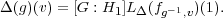The above equation can be turned around to define, for each L : HomG(V 1G,V 2G) a function ΔD.

Exercise 1.14. Show that if LHomG(V 1,V 2), then the function Δ : G HomC(V 1,V 2) defined by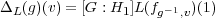is in D.

Exercise 1.15. Check that the maps ΔΔL and LLΔ are inverses of each other.

### 1.5. A criterion for irreducibility

Let G be a finite group, H a subgroup and (π,V ) a representation of H. The space V G can be decomposed into a direct sum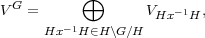where V Hx-1H consists of functions G V supported on Hx-1H: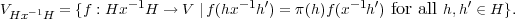V Hx-1H is stable under the action of π. Let πHx-1H denote the resulting representation of H on V Hx-1H and let xπHx-1Hx denote the representation of H xHx-1 on V given by xπ(h) = π(x-1hx).

Exercise 1.16. Show that f(hf(x-1h)) defines an isomorphism of representations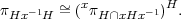We have proved

Proposition 1.17. Let G be a finite group and H any subgroup. For every representation π of H, there is a canonical isomorphism of representations of H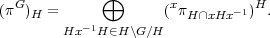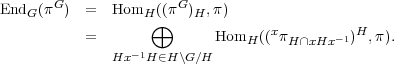Recall that πG is irreducible if and only if EndG(πG) is one dimensional. As a result, we obtain Mackey’s irreducibility criterion:

Theorem (Mackey’s irreducibility criterion). Let G be a finite group and H a subgroup. Let π be an irreducible representation of H. Then πG is irreducible if and only if, for any x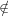H, the representations π and (xπHxHx-1)H are disjoint.

Corollary 1.18. Suppose that G is a finite group and H a normal subgroup. Then for any irreducible representation π of H, πG is irreducible if and only if for every xH, xπ is not isomorphic to π.

### 1.6. The little groups method

The little groups method was first used by Wigner [Wig39], and generalized by Mackey [Mac58] to construct representations of a group from those of a normal subgroup. We will restrict ourselves to the case where G is a finite group and N is a normal subgroup of G which is abelian. Let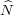denote the Pontryagin dual of N (Section 1.2). Define an action of G onby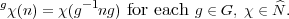Let ρ be an irreducible representation of G on the vector space V ρ. For each χ, write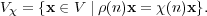Then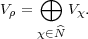Define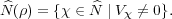Proposition 1.19 (Clifford’s theorem).(ρ) consists of a single G-orbit of.

Proof. Suppose xV χ, and gG. Then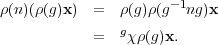Therefore,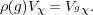(1.1)

It follows that gGV gχ is invariant under ρ. From the irreducibility of ρ one concludes that if V χ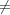0, then gGV gχ = V ρ.

For χ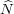(ρ), let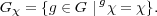It follows from (1.1) that for every gGχ, ρ(g) preserves V χ. Therefore, ρ gives rise to a representation ρχ of Gχ on V χ.

Proposition 1.20 (Mackey’s imprimitivity theorem).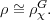Proof.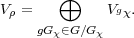Therefore, for each xV ρ, there is a unique decomposition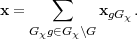By (1.1), ρ(g-1)xgGχV χ. The representation space of ρχG is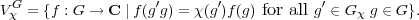Define φ(x)(g) = ρ(g)xg-1Gχ for each gG.

Exercise 1.21. Show that φ : V ρ ρχG is a well defined isomorphism of representations of G.